The aerodynamic theory of the lifting line was first solved by Multhopp1. This solution uses a spanwise distribution of the control points accordingly a sinus-function and is applicable to unswept wings. For the bell-shaped lift distribution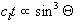a direct solution of the lifting line formula can be derived and is described here.

The integral for total lift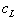can be written as (Truckenbrodt2):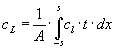(Tru. 5.42)

For a symetrical lift distribution this formula can be transformed: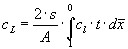with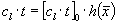;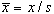(Equ. 00-01)

If the spanwise control points are aranged along a "central-angle"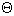similar to the Multhopp procedure, the following terms can be written:
 local lift load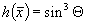and spanwise station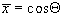(Mul. 07)

This leads to the final integration and its solution: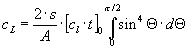with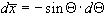(Equ. 00-02)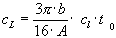(Equ. 00-03)

The total required geometrical twist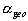is, accordingly to Truckenbrodt, the sum of effective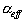and induced angle of attack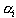. Angles are calculated in [rad.]: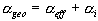(Tru 7.64)

The local effective angle of attack can be found with the term: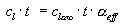(Tru. 7.11 & 7.28)

This term is now used in Equation (Equ. 00-01 b):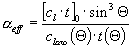(Equ. 00-04)

The solution for the induced angle of attack is more complex. Truckenbrodt gives the induced angle of attackfor unswept wings: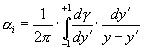with the circulation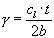(Tru. 7.67 & 7.70)

Using the following terms, we can transform this integrartion in the formulation as given in Multhopp: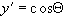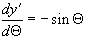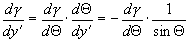(Equ. 00-05)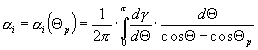(Mul. 13)

This formula now has to be solved for the bell shaped lift distribution: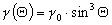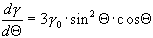(Equ. 00-06)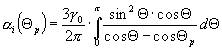(Equ. 00-07)

For this the term is split up in several parts, which can be integrated seperately. The calculation is described in the Appendix A1 . The final solution is: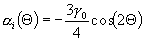or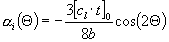(Equ. 00-08)

This gives the required calculation method for the geometrical twist, which can be transformed, using equation (Equ. 00-03):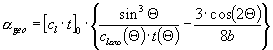(Equ. 00-09a)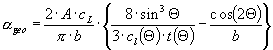(Equ. 00-09b)